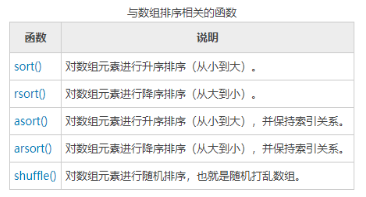• 已经成为了我们常用的网站编程语言，并且结合了C语言，Java等我们常见的编程语言，所以，有很多web开发领域的新人都看中了他的使用广泛性，有很多人都想了解php的内容，所以今天，我们就来讲讲PHP打乱数组顺序的方法...

【摘要】PHP作为一种超文本预处理器，已经成为了我们常用的网站编程语言，并且结合了C语言，Java等我们常见的编程语言，所以，有很多web开发领域的新人都看中了他的使用广泛性，有很多人都想了解php的内容，所以今天，我们就来讲讲PHP打乱数组顺序的方法。
shuffle() 函数用来随机打乱数组元素的顺序，其语法如下：
bool shuffle ( array &$arr ) 参数 arr 表示一个数组。 shuffle() 函数调用结束后， arr 数组元素的顺序会被打乱。 返回值：成功时返回 TRUE，失败时返回 FALSE。PHP打乱数组顺序的方法的使用示例如下：$info = array(
"url" => "http://c.biancheng.net/php/",
"language" => "PHP",
"update" => "2019-10-29",
"author" => "changsheng yan"
);
$num = array(20, 45, 8, 203, 3, 139, 48); shuffle($info);
print_r($info); shuffle($num);
print_r($num); ?> 执行以上PHP打乱数组顺序的方法程序的结果为：  展开全文•代码 • 主要介绍了JAVA随机打乱数组顺序的方法,包含了随机数的应用及数组的排序等操作,是Java操作数组的典型应用,需要的朋友可以参考下 • 已经成为了我们常用的网站编程语言，并且结合了C语言，Java等我们常见的编程语言，所以，有很多web开发领域的新人都看中了他的使用广泛性，有很多人都想了解php的内容，所以今天，我们就来讲讲PHP打乱数组顺序的方法...  【摘要】PHP作为一种超文本预处理器，已经成为了我们常用的网站编程语言，并且结合了C语言，Java等我们常见的编程语言，所以，有很多web开发领域的新人都看中了他的使用广泛性，有很多人都想了解php的内容，所以今天，我们就来讲讲PHP打乱数组顺序的方法。 shuffle() 函数用来随机打乱数组元素的顺序，其语法如下： bool shuffle ( array &$arr )
参数 arr 表示一个数组。
shuffle() 函数调用结束后， arr 数组元素的顺序会被打乱。
返回值：成功时返回 TRUE，失败时返回 FALSE。PHP打乱数组顺序的方法的使用示例如下：
$info = array( "url" => "http://c.biancheng.net/php/", "language" => "PHP", "update" => "2019-10-29", "author" => "changsheng yan" );$num = array(20, 45, 8, 203, 3, 139, 48);
shuffle($info); print_r($info);
shuffle($num); print_r($num);
?>
执行以上PHP打乱数组顺序的方法程序的结果为：
Array
(
 => changsheng yan
 => http://c.biancheng.net/php/
 => 2019-10-29
 => PHP
)
Array
(
 => 8
 => 139
 => 45
 => 3
 => 20
 => 203
 => 48
)
注意：因为是随机打乱，所以每次的执行结果会不一样。
以上就是有关于PHP打乱数组顺序的方法的相关内容了，作为一种超文本预处理器的常用语言，我相信已经有越来越多的人看到了他的价值，所以在个栏目里，小编会尽可能多的为大家分享有关于PHP的相关知识，当然，环球网校的小编也要在此祝大家的PHP学习之路顺利。

展开全文• ## java打乱数组顺序

万次阅读 2017-03-27 15:51:55
java打乱数组顺序

import java.util.*;    public class ShuffleTest {      public static void main(String[] args) {          List<Integer> list = new ArrayList<Integer>();          for (int i = 0; i < 10; i++)              list.add(new Integer(i));          System.out.println("打乱前:");          System.out.println(list);            for (int i = 0; i < 5; i++) {              System.out.println("第" + i + "次打乱：");              Collections.shuffle(list);              System.out.println(list);          }      }  }    参考：http://blog.csdn.net/zzqkillyou/article/details/7388690

游戏中遇到这样的问题，需要将一组已知的数据打乱，按照以前和现在的做法，总结了以下方法。
方法一，最笨的菜鸟方法，也是容易想到的（幸好我没想过这种方法 :)）
从已知数组中随机一个数，然后加入到另一个数组中，在加入之前，先检查是否已经加入过。
这种方法有很大运气成分，且数据越大，效率越低，超过一定数目，则程序几乎无法执行，会一直卡在那里，代码：

[java]
view plain
copy

package com.test;    import java.util.Random;      public class TestArray {   public static int runCount =0;//用于记录方法运算次数      public int []  m1(int [] arr){    Random ran = new Random();    int length = arr.length;    int [] newArr = new int[length];    int count =0;    while (true) {     runCount ++;     int r = ran.nextInt(length)+1;     if(!exist(r, newArr)){      newArr [count] = r;      count++;     }     if(count==length){      break;     }    }    System.out.println("m1 运算次数  = "+runCount);    return newArr;   }      public static boolean exist(int a,int [] arr){    for (int i = 0; i < arr.length; i++) {     if(arr[i] ==a){      return true;     }    }    return false;   }

方法二，这种方法是我遇到这个问题就想到的，思路就是：先随机出已知数组的下标值，然后取出这个数放到另一个数组中，再从已知数组中删除这个数。这种方法的运算次数是根据数组长度而定的，缺点是 大部分运算都耗在删除的判断上，主要是因为 数组不像list那样，能删除一个指定位置的值。代码：

[java]
view plain
copy

//-----------------------------------------------  public int []  m2(int [] arr){   Random ran = new Random();   int [] newArr = new int[arr.length];   int k = 0;   while (arr.length>0) {    runCount++;    int index = ran.nextInt(arr.length);//随即数组下标    newArr[k++] = arr[index];    arr = removeElement(arr[index], arr);   }   System.out.println("m2运算次数  = "+runCount);   return newArr;  }    public int [] removeElement(int ele,int [] arr){   int [] arr2 =new int[arr.length-1];   int k = 0;   for (int i = 0; i < arr.length; i++) {    runCount++;    if(arr[i]!=ele){     arr2[k++] = arr[i];    }   }   return arr2;  }

方法三， 这种方法就很巧妙了，是在c 的代码中看到，翻译成了Java代码，它的巧妙在于：每次从已知数组随机一个数，然后将数组的最后一个值 赋值给前面随机到的数的位置上，然后将长度-1，再从原数组下标-1的数组中随机。 运算次数就是数组长度，这种方法真的很聪明啊，以下是代码：

[java]
view plain
copy

//-----------------------------------------------  public int []  m3(int [] arr) {   int [] arr2 =new int[arr.length];   int count = arr.length;   int cbRandCount = 0;// 索引   int cbPosition = 0;// 位置   int k =0;   do {    runCount++;    Random rand = new Random();    int r = count - cbRandCount;    cbPosition = rand.nextInt(r);     arr2[k++] = arr[cbPosition];    cbRandCount++;    arr[cbPosition] = arr[r - 1];// 将最后一位数值赋值给已经被使用的cbPosition   } while (cbRandCount < count);   System.out.println("m3运算次数  = "+runCount);   return arr2;  }

测试下代码：

[java]
view plain
copy

// ----------------------------------------------    public static void main(String[] args) {      int[] arr = new int;      for (int i = 0; i < arr.length; i++) {          arr[i] = i + 1;      }      TestArray t = new TestArray();      arr = t.m1(arr);      print(arr);      arr = t.m2(arr);      print(arr);      arr = t.m3(arr);      print(arr);  }    public static void print(int[] arr) {      for (int i = 0; i < arr.length; i++) {          System.out.print(arr[i] + " ");      }      System.out.println();      runCount = 0;  }

结果：

[java]
view plain
copy

m1 运算次数  = 51  3 10 2 1 5 9 6 8 4 7   m2运算次数  = 65  1 3 8 2 10 5 9 6 7 4   m3运算次数  = 10  10 7 8 3 1 5 6 9 2 4

第三种方法还是值得学习的，大家如果有其它好的方法和思路欢迎补充~~

展开全文• 今天小编就为大家分享一篇对Python random模块打乱数组顺序的实例讲解，具有很好的参考价值，希望对大家有所帮助。一起跟随小编过来看看吧
• 主要介绍了JavaScript随机打乱数组顺序之随机洗牌算法的相关资料,非常不错，具有参考借鉴价值，需要的朋友可以参考下
• // 打乱数组顺序 function update_sort(testArray){ if (!Array.prototype.derangedArray) { Array.prototype.derangedArray = function() { for(var j, x, i = this.length; i; j = parseInt(Math.random...
	// 打乱数组顺序
function update_sort(testArray){

if (!Array.prototype.derangedArray) {

Array.prototype.derangedArray = function() 
展开全文• ## 打乱数组顺序的三种方法

万次阅读 热门讨论 2018-03-16 11:20:12
sort排序法（最简单的打乱数组顺序的方法） 原理： 利用sort用法：arr.sort(compareFunction) 如果 compareFunction(a,b) 返回的值大于 0 ，则 b 在 a 的前边； 如果 compareFunction(a,b) 返回的值...
• 最近在做一个考试小程序的Api接口，其中一个是需要将试题列表打乱顺序，都知道，从数据库搜索出来的列表通常为数组的形式，所以我面临的问题就变成了 如何打乱数组顺序。 随手一百度，shuffle()函数 :把片段中的...php
• /*打乱数组顺序*/ var tempArray = [0,1,2,3,4]; tempArray.sort(function(a,b){ /*用Math.random()函数生成0~1之间的随机数与0.5比较，返回-1或1*/ return Math.random()>0.5 ...javascript js
• random.shuffle()函数可以随机打乱数组顺序 import random random.shuffle(x1)
• //每一次的遍历都相当于把从数组中随机抽取（不重复）的一个元素放到数组的最后面（索引顺序为：len-1,len-2,len-3......0） return arr; } 　 转载于:https://www.cnblogs.com/YAN-HUA/p/9430326.html
• function randomsort(a, b) { return Math.random() > .5 ? -1 : 1; } this.questionArray.sort(randomsort)前段 mpvue vue
• ## C#打乱数组顺序

千次阅读 2018-11-15 11:49:49
//随机打算int数组 public int[] GetRandomNum(int[] num) { for (int i = 0; i &lt; num.Length; i++) { int temp = num[i]; int randomIndex = Random.Range(0, num.Length...unity
• 在我们使用一些数据的过程中，我们想要打乱数组内数据的顺序但不改变数据本身，可以通过改变索引值来实现，也就是将索引值重新随机排列，然后生成新的数组。功能主要由python中random模块的sample()函数实现。sample...python random 索引 数据...